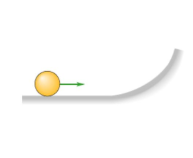# Problem: Four objects of various masses, each denoted m, all have the same radius. They are all rolling at the same speed as they approach a curved incline as shown below. Rank the objects based on the maximum height they reach along the curved incline.Solid disk, m = 0.5 kgHollow sphere, m = 0.2 kghoop, m = 0.2 kgSolid cylinder, m = 0.2 kgsolid sphere, m = 1.0 kg

###### FREE Expert Solution

Moment of inertia of the objects:

Solid sphere:

$\overline{){\mathbf{I}}{\mathbf{=}}\frac{\mathbf{2}}{\mathbf{5}}{\mathbf{M}}{{\mathbf{R}}}^{{\mathbf{2}}}}$

Solid cylinder/disk:

$\overline{){\mathbf{I}}{\mathbf{=}}\frac{\mathbf{1}}{\mathbf{2}}{{\mathbf{MR}}}^{{\mathbf{2}}}}$

Hoop:

$\overline{){\mathbf{I}}{\mathbf{=}}{{\mathbf{MR}}}^{{\mathbf{2}}}}$

Thin spherical shell/hollow sphere:

$\overline{){\mathbf{I}}{\mathbf{=}}\frac{\mathbf{2}}{\mathbf{3}}{{\mathbf{MR}}}^{{\mathbf{2}}}}$

Gravitational potential energy:

$\overline{){\mathbf{U}}{\mathbf{=}}{\mathbf{m}}{\mathbf{g}}{\mathbf{h}}}$

The kinetic energy of a rolling object:

$\overline{){\mathbf{K}}{\mathbf{E}}{\mathbf{=}}\frac{\mathbf{1}}{\mathbf{2}}{\mathbf{m}}{{\mathbf{v}}}^{{\mathbf{2}}}{\mathbf{+}}\frac{\mathbf{1}}{\mathbf{2}}{\mathbit{I}}{{\mathbf{\omega }}}^{{\mathbf{2}}}}$

Angular frequency:

$\overline{){\mathbf{\omega }}{\mathbf{=}}\frac{\mathbf{v}}{\mathbf{r}}}$

Conservation of energy:

88% (130 ratings)###### Problem Details

Four objects of various masses, each denoted m, all have the same radius. They are all rolling at the same speed as they approach a curved incline as shown below.Rank the objects based on the maximum height they reach along the curved incline.

Solid disk, m = 0.5 kg

Hollow sphere, m = 0.2 kg

hoop, m = 0.2 kg

Solid cylinder, m = 0.2 kg

solid sphere, m = 1.0 kg